We have seen in my previous blog post how `CALCULATE` can be used to modify the filter context of an expression. But, `CALCULATE` also performs another important task, this is called context transition.

## What is context transition?

If `CALCULATE` is executed inside of a row context, `CALCULATE` will take that row context and transform it into an equivalent filter context. `CALCULATE` will then apply this filter context to the data model, before computing its expression.

We can demonstrate context transition in action within a simple Power BI report. If we use the following expression to create a new calculated column in the 'Product' table:

``````Grand Total Unit Price = SUM ( 'Product' [Unit Price] )
``````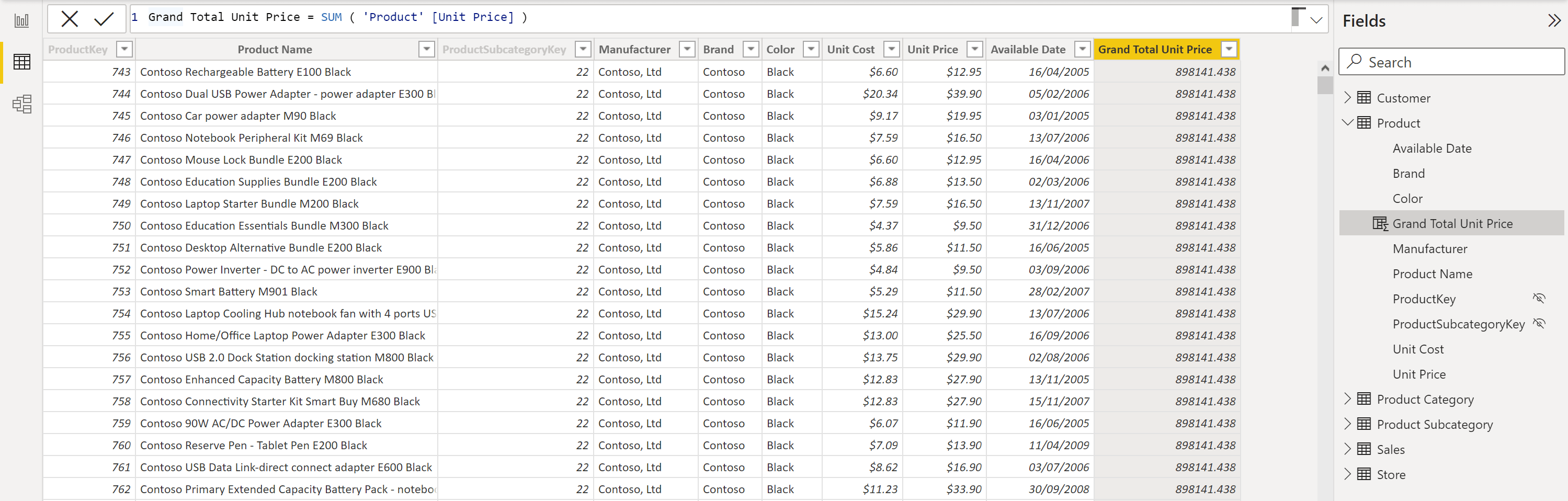We can see that the new calculated column contains the same value in every row. This value is the grand total of all unit prices. This is because `SUM` aggregates all values which are visible in the current filter context. As the filter context is currently empty, we are not filtering the 'Product' table, `SUM` has aggregated all of the rows in the 'Unit Price' column, and now this value is displayed in every row.

Now, let's modify this calculated column and wrap the expression in a `CALCULATE` function:

``````Unit Price CALCULATE = CALCULATE ( SUM ( 'Product' [Unit Price] ) )
``````

As we are creating a calculated column here, we automatically have a row context defined. And as we know, if `CALCULATE` is executed inside of a row context, it can perform context transition, transforming the row context (i.e. the current row) into an equivalent filter context.

This means that the expression: `SUM ( 'Product' [Unit Price] )` will be executed in a filter context that contains only the currently iterated row. We can now see that for each row, the result is no longer the grand total of unit price, but instead will be the unit price of the current product only. We can verify this, as for each product, the result in the 'Unit Price CALCULATE' column matches the value in the 'Unit Price' column. Here, context transition has been able to completely change the way a simple `SUM` is being executed.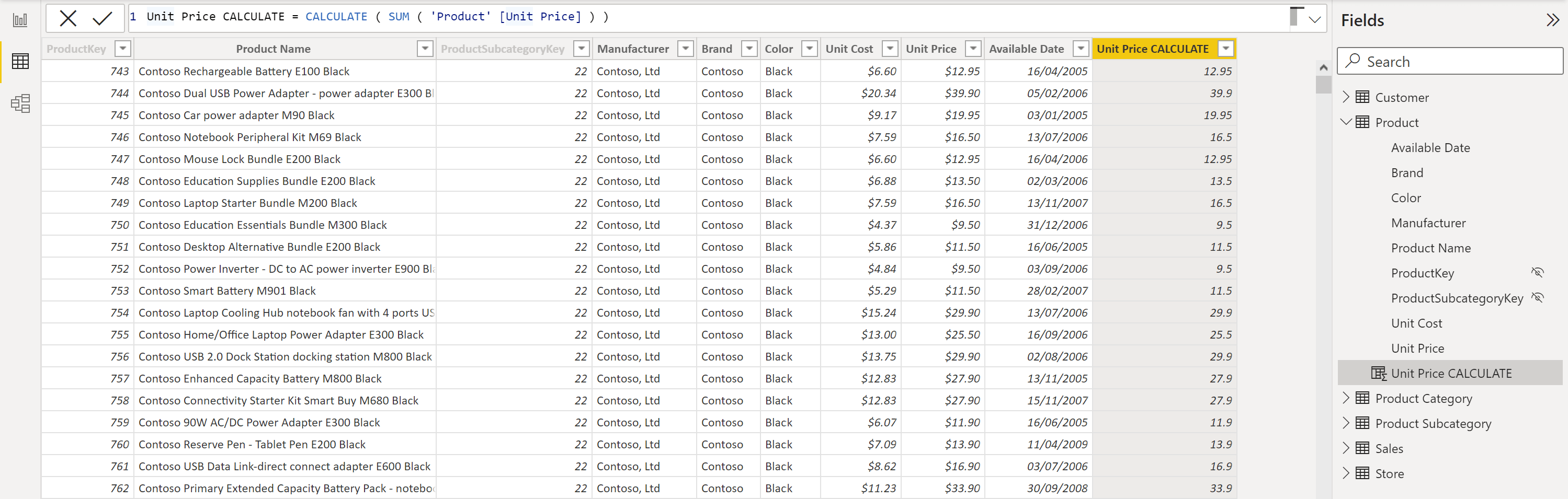## Evaluation contexts and relationships

In order to understand the second part of this blog post, it is important to take a look at how evaluation contexts and relationships in the data model interact. Relationships affect the way the filter context and the row context impact the calculation of a DAX expression.

For row contexts, the rule is simple. Row contexts never propagate through relationships. So starting from the current row, we cannot access columns which are coming from other related tables. To do this, we would need to be explicit, using `RELATED` or `RELATEDTABLE` functions (as explained in my previous blog post on this topic).

However, the filter context is more interesting. The rule is: the filter context propagates through relationships in the data model, moving from the one-side to the many-side of the relationship, but not in the opposite direction.

So if we look at the relationship in our data model between the 'Sales' and the 'Product' table, we can see that the relationship has a direction - one-to-many. So the filter context propagates from the 'Products' table (on the one-side) to the 'Sales' table (on the many-side) but not in the other direction.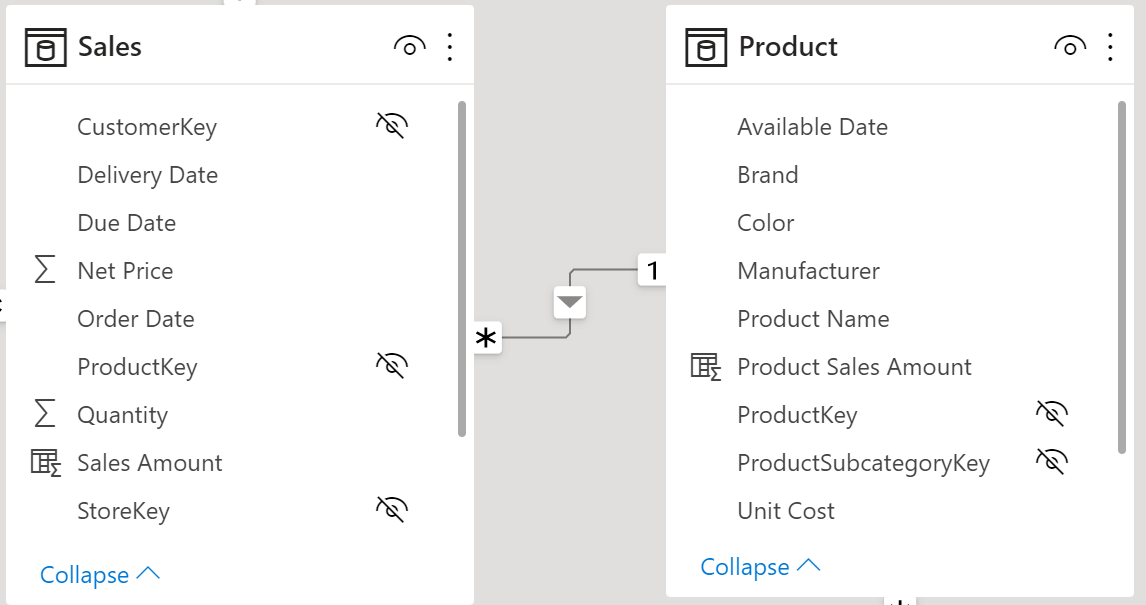## Context transition and relationships

Now, let’s create another calculated column in the 'Product' table, but instead we will compute on the 'Sales' table:

``````Grand Total Sales Amount = SUM ( Sales[Sales Amount] )
``````

This will compute the `SUM` of the 'Sales Amount' column for all of the rows which are currently visible in the current filter context. The filter context, right now, on the 'Sales' table is empty. We are not filtering the 'Sales' table. So, we will see that the value for each row, again is always the same. It is the grand total of all sales.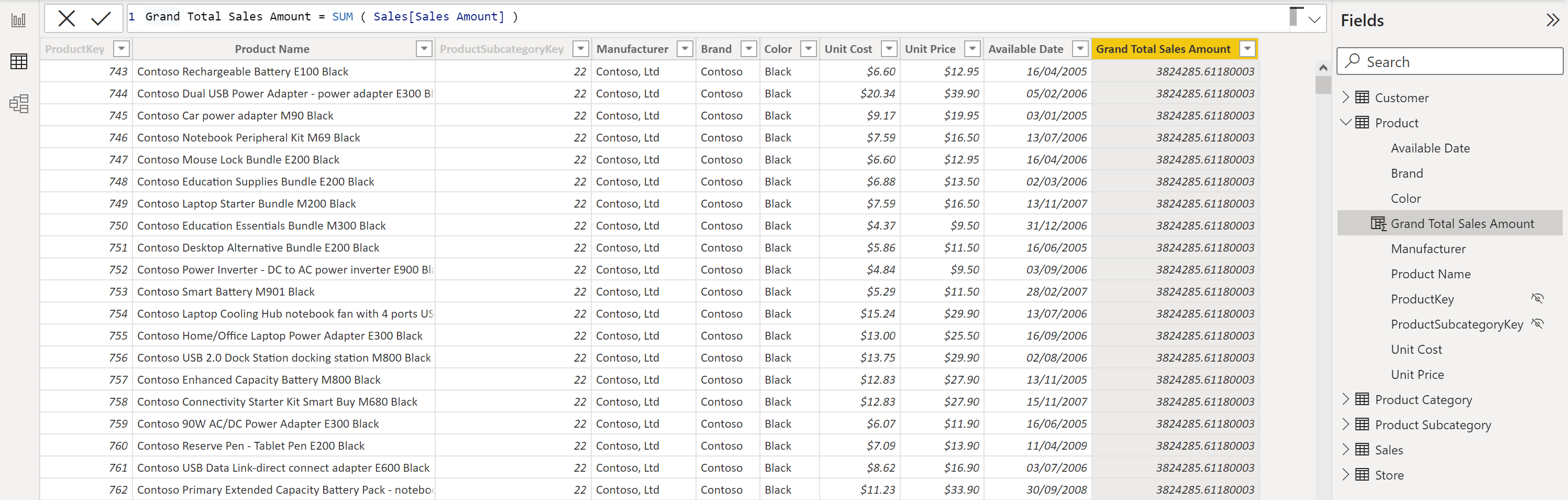Now just as we did before, let's wrap the expression in a `CALCULATE` and explore how this affects the result:

``````Sales Amount CALCULATE  = CALCULATE ( SUM ( Sales[Sales Amount] ) )
``````

So for each row, we start with the row context i.e. the current row. `CALCULATE` performs context transition, meaning that for each row, the row context has been transformed into a filter context, containing a single product. Now this filter context can propagate through relationships in the data model, and starting from the 'Product' table (on the one-side), it will be able to reach the 'Sales' table (on the many-side).

In other words, the filter context of a single product, can reach 'Sales', and the result will no longer be the grand total of all sales, but instead will be the sales of the current product only.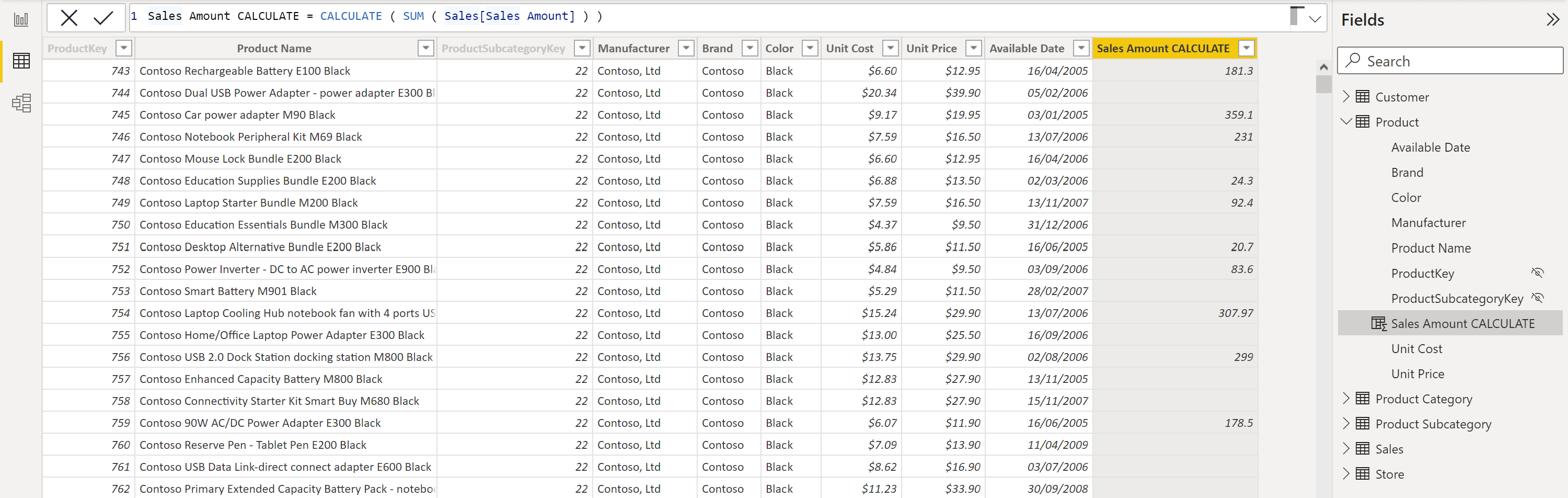## Automatic CALCULATE for measures

If we create a new measure:

``````Total Sales Amount = SUM ( Sales[Sales Amount] )
``````

Then we create a new calculated column in the 'Product' table:

``````Product Sales Amount = [Total Sales Amount]
``````As there is no `CALCULATE` function here, we would expect that this measure would compute the grand total of all sales for all of the rows. However, the result is not what we would expect. It is as though `CALCULATE` is there, doing a context transition and returning the sales amount for only the current product. This is exactly what is happening.

The rule is whenever a measure is called from inside a DAX expression, that measure is automatically surrounded with a `CALCULATE`. To illustrate this, the below expressions are equivalent:

``````Product Sales Amount = [Total Sales Amount]

Product Sales Amount = CALCULATE ( [Total Sales Amount] )
``````

This is extremely important to know, because we have seen how `CALCULATE` completely changes the result we are computing.

This is why, by convention, we do not reference table names in front of measures. As when we read the DAX code, we need to immediately recognise it as being measure. This way, we know `CALCULATE` is there, performing context transition and doing its job.

## Conclusion

In this blog post, we have seen that context transition is a powerful property of `CALCULATE`. When used inside of a row context, `CALCULATE` transitions that row context into a corresponding filter context. This filter context can then propagate through relationships in the data model from the one-side, to the many-side. Finally, we have seen how when measures are used inside of DAX expressions, they are automatically wrapped in a `CALCULATE`. Understanding this behaviour allows us to avoid unexpected results when using measures inside of calculations.

### Jessica Hill

#### Apprentice Engineer IIIJessica comes from a Biosciences background, having gained a 1st Class Bachelor of Science in Biology from The University of Manchester.

During the lockdown Jessica used the opportunity to explore her interest in technology by taking two Code First Girls online courses; Introduction to Web Development and Python Programming.

This led Jessica to look for job opportunities in the technology sector and joined endjin's 2021 apprenticeship cohort, which had over 200 applicants.